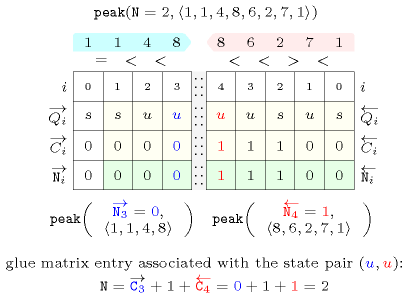## 5.318. peak

Origin
Constraint

$\mathrm{𝚙𝚎𝚊𝚔}\left(𝙽,\mathrm{𝚅𝙰𝚁𝙸𝙰𝙱𝙻𝙴𝚂}\right)$

Arguments
 $𝙽$ $\mathrm{𝚍𝚟𝚊𝚛}$ $\mathrm{𝚅𝙰𝚁𝙸𝙰𝙱𝙻𝙴𝚂}$ $\mathrm{𝚌𝚘𝚕𝚕𝚎𝚌𝚝𝚒𝚘𝚗}\left(\mathrm{𝚟𝚊𝚛}-\mathrm{𝚍𝚟𝚊𝚛}\right)$
Restrictions
 $𝙽\ge 0$ $2*𝙽\le \mathrm{𝚖𝚊𝚡}\left(|\mathrm{𝚅𝙰𝚁𝙸𝙰𝙱𝙻𝙴𝚂}|-1,0\right)$ $\mathrm{𝚛𝚎𝚚𝚞𝚒𝚛𝚎𝚍}$$\left(\mathrm{𝚅𝙰𝚁𝙸𝙰𝙱𝙻𝙴𝚂},\mathrm{𝚟𝚊𝚛}\right)$
Purpose

A variable ${V}_{k}$ $\left(1 of the sequence of variables $\mathrm{𝚅𝙰𝚁𝙸𝙰𝙱𝙻𝙴𝚂}={V}_{1},\cdots ,{V}_{m}$ is a peak if and only if there exists an $i$ (with $1) such that ${V}_{i-1}<{V}_{i}$ and ${V}_{i}={V}_{i+1}=\cdots ={V}_{k}$ and ${V}_{k}>{V}_{k+1}$. $𝙽$ is the total number of peaks of the sequence of variables $\mathrm{𝚅𝙰𝚁𝙸𝙰𝙱𝙻𝙴𝚂}$.

Example
 $\left(2,〈1,1,4,8,6,2,7,1〉\right)$ $\left(0,〈1,1,4,4,4,6,7,7〉\right)$ $\left(4,〈1,5,4,9,4,6,2,7,6〉\right)$

The first $\mathrm{𝚙𝚎𝚊𝚔}$ constraint holds since the sequence $11486271$ contains two peaks that respectively correspond to the variables that are assigned to values 8 and 7.

##### Figure 5.318.1. Illustration of the first example of the Example slot: a sequence of eight variables ${V}_{1}$, ${V}_{2}$, ${V}_{3}$, ${V}_{4}$, ${V}_{5}$, ${V}_{6}$, ${V}_{7}$, ${V}_{8}$ respectively fixed to values 1, 1, 4, 8, 6, 2, 7, 1 and its corresponding two peaks ($𝙽=2$)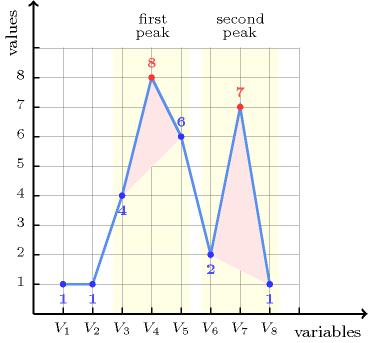All solutions

Figure 5.318.2 gives all solutions to the following non ground instance of the $\mathrm{𝚙𝚎𝚊𝚔}$ constraint: $𝙽\in \left[1,2\right]$, ${V}_{1}\in \left[1,2\right]$, ${V}_{2}=2$, ${V}_{3}\in \left[1,2\right]$, ${V}_{4}\in \left[1,2\right]$, ${V}_{5}\in \left[2,3\right]$, $\mathrm{𝚙𝚎𝚊𝚔}$$\left(𝙽,〈{V}_{1},{V}_{2},{V}_{3},{V}_{4},{V}_{5}〉\right)$.

##### Figure 5.318.2. All solutions corresponding to the non ground example of the $\mathrm{𝚙𝚎𝚊𝚔}$ constraint of the All solutions slot where each peak is coloured in orangeTypical
 $|\mathrm{𝚅𝙰𝚁𝙸𝙰𝙱𝙻𝙴𝚂}|>2$ $\mathrm{𝚛𝚊𝚗𝚐𝚎}$$\left(\mathrm{𝚅𝙰𝚁𝙸𝙰𝙱𝙻𝙴𝚂}.\mathrm{𝚟𝚊𝚛}\right)>1$
Symmetries
• Items of $\mathrm{𝚅𝙰𝚁𝙸𝙰𝙱𝙻𝙴𝚂}$ can be reversed.

• One and the same constant can be added to the $\mathrm{𝚟𝚊𝚛}$ attribute of all items of $\mathrm{𝚅𝙰𝚁𝙸𝙰𝙱𝙻𝙴𝚂}$.

Arg. properties
• Functional dependency: $𝙽$ determined by $\mathrm{𝚅𝙰𝚁𝙸𝙰𝙱𝙻𝙴𝚂}$.

• Contractible wrt. $\mathrm{𝚅𝙰𝚁𝙸𝙰𝙱𝙻𝙴𝚂}$ when $𝙽=0$.

Usage

Useful for constraining the number of peaks of a sequence of domain variables.

Remark

Since the arity of the arc constraint is not fixed, the $\mathrm{𝚙𝚎𝚊𝚔}$ constraint cannot be currently described with the graph-based representation. However, this would not hold anymore if we were introducing a slot that specifies how to merge adjacent vertices of the final graph.

Counting
 Length ($n$) 2 3 4 5 6 7 8 Solutions 9 64 625 7776 117649 2097152 43046721

Number of solutions for $\mathrm{𝚙𝚎𝚊𝚔}$: domains $0..n$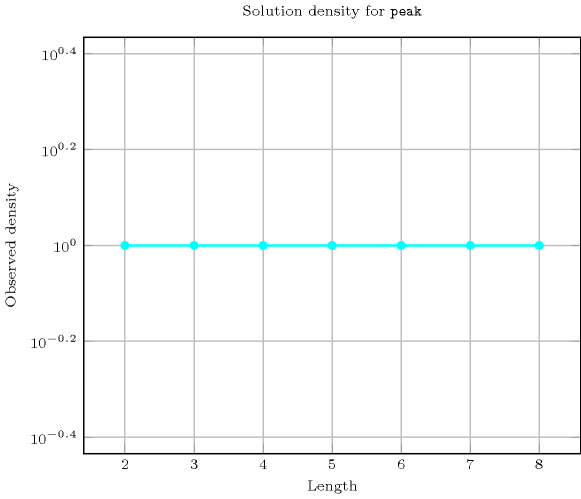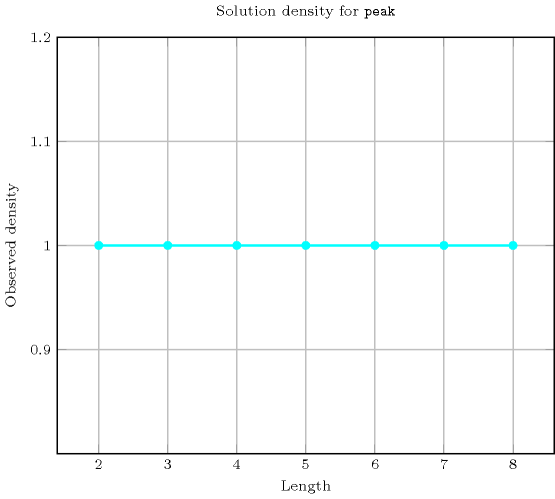Length ($n$)2345678
Total9646257776117649209715243046721
 Parameter value

095029517921108869498439791
1-1433053137352894443011654622
2---67133033101092224895038
3-----723026057270

Solution count for $\mathrm{𝚙𝚎𝚊𝚔}$: domains $0..n$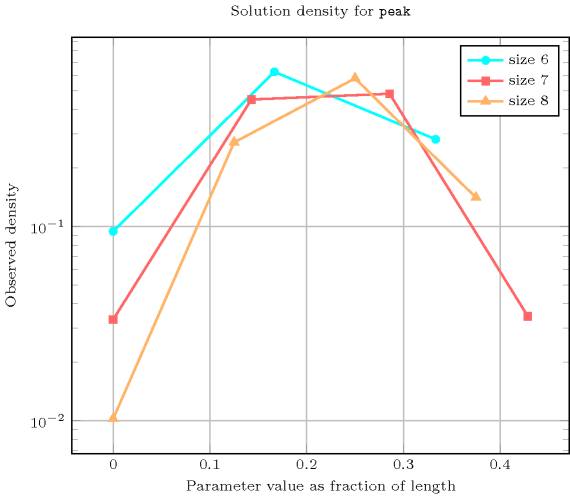generalisation: $\mathrm{𝚋𝚒𝚐}_\mathrm{𝚙𝚎𝚊𝚔}$ (a tolerance parameter is added for counting only big peaks).

specialisation: $\mathrm{𝚗𝚘}_\mathrm{𝚙𝚎𝚊𝚔}$ (the variable counting the number of peaks is set to 0 and removed).

Keywords
Cond. implications

$•$ $\mathrm{𝚙𝚎𝚊𝚔}\left(𝙽,\mathrm{𝚅𝙰𝚁𝙸𝙰𝙱𝙻𝙴𝚂}\right)$

with  $𝙽>0$

implies $\mathrm{𝚊𝚝𝚕𝚎𝚊𝚜𝚝}_\mathrm{𝚗𝚟𝚊𝚕𝚞𝚎}$$\left(\mathrm{𝙽𝚅𝙰𝙻},\mathrm{𝚅𝙰𝚁𝙸𝙰𝙱𝙻𝙴𝚂}\right)$

when  $\mathrm{𝙽𝚅𝙰𝙻}=2$.

$•$ $\mathrm{𝚙𝚎𝚊𝚔}\left(𝙽,\mathrm{𝚅𝙰𝚁𝙸𝙰𝙱𝙻𝙴𝚂}\right)$

implies $\mathrm{𝚒𝚗𝚏𝚕𝚎𝚡𝚒𝚘𝚗}$$\left(𝙽,\mathrm{𝚅𝙰𝚁𝙸𝙰𝙱𝙻𝙴𝚂}\right)$

when  $𝙽=\mathrm{𝚙𝚎𝚊𝚔}\left(\mathrm{𝚅𝙰𝚁𝙸𝙰𝙱𝙻𝙴𝚂}.\mathrm{𝚟𝚊𝚛}\right)+$$\mathrm{𝚟𝚊𝚕𝚕𝚎𝚢}$$\left(\mathrm{𝚅𝙰𝚁𝙸𝙰𝙱𝙻𝙴𝚂}.\mathrm{𝚟𝚊𝚛}\right)$.

Automaton

Figure 5.318.3 depicts the automaton associated with the $\mathrm{𝚙𝚎𝚊𝚔}$ constraint. To each pair of consecutive variables $\left({\mathrm{𝚅𝙰𝚁}}_{i},{\mathrm{𝚅𝙰𝚁}}_{i+1}\right)$ of the collection $\mathrm{𝚅𝙰𝚁𝙸𝙰𝙱𝙻𝙴𝚂}$ corresponds a signature variable ${S}_{i}$. The following signature constraint links ${\mathrm{𝚅𝙰𝚁}}_{i}$, ${\mathrm{𝚅𝙰𝚁}}_{i+1}$ and ${S}_{i}$: $\left({\mathrm{𝚅𝙰𝚁}}_{i}<{\mathrm{𝚅𝙰𝚁}}_{i+1}⇔{S}_{i}=0\right)\wedge \left({\mathrm{𝚅𝙰𝚁}}_{i}={\mathrm{𝚅𝙰𝚁}}_{i+1}⇔{S}_{i}=1\right)\wedge \left({\mathrm{𝚅𝙰𝚁}}_{i}>{\mathrm{𝚅𝙰𝚁}}_{i+1}⇔{S}_{i}=2\right)$.

##### Figure 5.318.3. Automaton of the $\mathrm{𝚙𝚎𝚊𝚔}$ constraint##### Figure 5.318.4. Hypergraph of the reformulation corresponding to the automaton of the $\mathrm{𝚙𝚎𝚊𝚔}$ constraint (since all states of the automaton are accepting there is no restriction on the last variable ${Q}_{n-1}$)##### Figure 5.318.5. Glue matrix of the $\mathrm{𝚙𝚎𝚊𝚔}$ constraint
 $s$ $\left({\left\{>|=\right\}}^{*}\right)$ $u$ $\left(<{\left\{<|=\right\}}^{*}\right)$ $s$ $\left({\left\{>|=\right\}}^{*}\right)$ $\stackrel{\to }{C}+\stackrel{←}{C}$ $\stackrel{\to }{C}+\stackrel{←}{C}$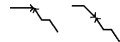$u$ $\left(<{\left\{<|=\right\}}^{*}\right)$ $\stackrel{\to }{C}+\stackrel{←}{C}$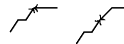$\stackrel{\to }{C}+1+\stackrel{←}{C}$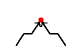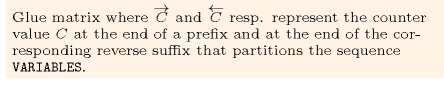##### Figure 5.318.6. Illustrating the use of the state pair $\left(u,u\right)$ of the glue matrix for linking $𝙽$ with the counters variables obtained after reading the prefix $1,1,4,8$ and corresponding suffix $8,6,2,7,1$ of the sequence $1,1,4,8,6,2,7,1$; note that the suffix $8,6,2,7,1$ (in pink) is proceed in reverse order; the left (resp. right) table shows the initialisation (for $i=0$) and the evolution (for $i>0$) of the state of the automaton and of its counter $C$ upon reading the prefix $1,1,4,8$ (resp. the suffix $1,7,2,6,8$).